IF YOU CAN SEE THIS go to /sfa/Nuprl/Shared/Xindentation_hack_doc.html

### Integers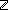is the type of integers; e.g.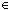and -1.

The usual arithmetic operators that are primitive in this system are these:

-a
a+b
a-b
a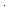b
a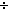b
a rem b

The following comparison operators happen also to be primitive in this system:

if a=b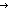s ; t fi
if a<bs ; t fi
a<b

The two "conditionals" simply compare integers and take the appropriate branch. The "a<b" form represents the less-than relation on integers. See Propositions.

if 0=0s ; t fi *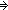s
if 0=1s ; t fi *t
if 0<1s ; t fi *s
if 0<0s ; t fi *t

There are also some often-used Boolean expressions for these integer relations:

Def i=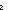j == if i=jtrue; falsefi
Def i<j == if i<jtrue; falsefi
Def i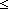j ==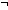j<i

Several Subtypes ofare in common use.

Def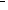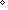== {i:i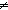0 }
Def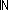== {i:| 0i }
Def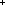== {i:| 0<i }
Def {i...j} == {k:ik & kj }
Def {i..j} == {k:ik < j }

"k" is an abbreviated display for {0..k}, which has been adopted as Nuprl's standard finite type with kelements.

Integer Literals

In Nuprl the canonical form for an integer is atomic, rather than, say, bit strings or iteration of successor from zero. There is a small complication involving the implementation of negative literals, which will be of interest only to those interested in reconciling the implementation with theory. See Negative Literals.

(March 2001 - sfa )